## Two spherical inflated rubber balloons each have the same amount of charge spread uniformly on their surfaces. If the repelling force is 2.5

Question

Two spherical inflated rubber balloons each have the same amount of charge spread uniformly on their surfaces. If the repelling force is 2.5 N and the distance between the balloon centers is 0.30 m, find how much charge is on each balloon.

in progress 0
2 months 2021-07-31T22:25:30+00:00 1 Answers 3 views 0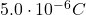Explanation:

The magnitude of the electrostatic force between two charged objects is given by Coulomb’s law: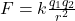where: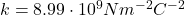is the Coulomb’s constant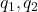are the two charges  on the two objects

r is the separation between the two charges

The force is:

– Repulsive if the two charges have same sign

– Attractive if the two charges have opposite sign

In this problem:

F = 2.5 N is the force between the two balloons

r = 0.30 m is their separation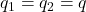is the charge on each balloon (they have the same charge)

So, re-arranging the equation, we can find the value of q: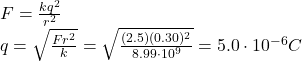We are also told that the force between them is repulsive: this means that the charges on the two balloons have  same sign (so, either they are both positive, or both negative).